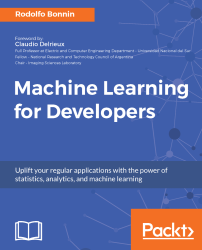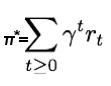•#### Machine Learning for Developers#### Overview of this book

Most of us have heard about the term Machine Learning, but surprisingly the question frequently asked by developers across the globe is, “How do I get started in Machine Learning?”. One reason could be attributed to the vastness of the subject area because people often get overwhelmed by the abstractness of ML and terms such as regression, supervised learning, probability density function, and so on. This book is a systematic guide teaching you how to implement various Machine Learning techniques and their day-to-day application and development. You will start with the very basics of data and mathematical models in easy-to-follow language that you are familiar with; you will feel at home while implementing the examples. The book will introduce you to various libraries and frameworks used in the world of Machine Learning, and then, without wasting any time, you will get to the point and implement Regression, Clustering, classification, Neural networks, and more with fun examples. As you get to grips with the techniques, you’ll learn to implement those concepts to solve real-world scenarios for ML applications such as image analysis, Natural Language processing, and anomaly detections of time series data. By the end of the book, you will have learned various ML techniques to develop more efficient and intelligent applications.
PrefaceFree Chapter
Introduction - Machine Learning and Statistical ScienceThe Learning ProcessClusteringLinear and Logistic RegressionNeural NetworksConvolutional Neural NetworksRecurrent Neural NetworksRecent Models and DevelopmentsSoftware Installation and Configuration# Basic RL techniques: Q-learning

One of the most well-known reinforcement learning techniques, and the one we will be implementing in our example, is Q-learning.

Q-learning can be used to find an optimal action for any given state in a finite Markov decision process. Q-learning tries to maximize the value of the Q-function that represents the maximum discounted future reward when we perform action a in state s.

Once we know the Q-function, the optimal action a in state s is the one with the highest Q-value. We can then define a policy π(s), that gives us the optimal action in any state, expressed as follows:We can define the Q-function for a transition point (st, at, rt, st+1) in terms of the Q-function at the next point (st+1, at+1, rt+1, st+2), similar to what we did with the total discounted future reward. This equation is known as the Bellman equation for Q-learning...